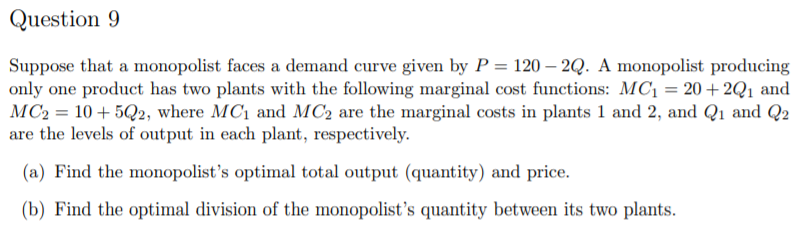# Please answer me in detail. Thank you. Question 9 Suppose that a monopolist faces a demand curve given by P 120-2Q. A m...Question 9 Suppose that a monopolist faces a demand curve given by P 120-2Q. A monopolist producing only one product has two plants with the following marginal cost functions: MC1 20+2Q1 and MC2-10+502, where MCi and MC2 are the marginal costs in plants 1 and 2, and Q1 and Q2 are the levels of output in each plant, respectively. (a) Find the monopolist's optimal total output (quantity) and price. b) Find the optimal division of the monopolist's quantity between its two plants.

P = 120 - 2Q

(a)

Total revenue (TR) = P x Q = 120Q - 2Q2

Marginal revenue (MR) = dTR/dQ = 120 - 4Q

MC1 = 20 + 2Q1, therefore Q1 = (MC1 - 20) / 2 = 0.5MC1 - 10

MC2 = 10 + 5Q2, therefore Q2 = (MC2 - 10) / 5 = 0.2MC2 - 2

As Q = Q1 + Q2,

Q = 0.5MC1 - 10 + 0.2MC2 - 2

Q = 0.5MC1 + 0.2MC2 - 12

Equating MC1 and MC2 as MC,

Q = 0.5MC + 0.2MC - 12

Q = 0.7MC - 12

0.7MC = Q + 12

MC = (Q + 12) / 0.7

Profit-maximizing condition is: MR = MC.

120 - 4Q = (Q + 12) / 0.7

84 - 2.8Q = Q + 12

3.8Q = 72

Q = 18.95

P = 120 - (2 x 18.95) = 120 - 37.9 = 82.1

(b)

MC = (Q + 12) / 0.7 = (18.95 + 12) / 0.7 = 30.95 / 0.7 = 44.21

Q1 = 0.5MC - 10 = (0.5 x 44.21) - 10 = 22.11 - 10 = 12.11

Q2 = 0.2MC - 2 = (0.2 x 44.21) - 2 = 8.84 - 2 = 6.84

##### Add Answer of: Please answer me in detail. Thank you. Question 9 Suppose that a monopolist faces a demand curve given by P 120-2Q. A m...
More Homework Help Questions Additional questions in this topic.